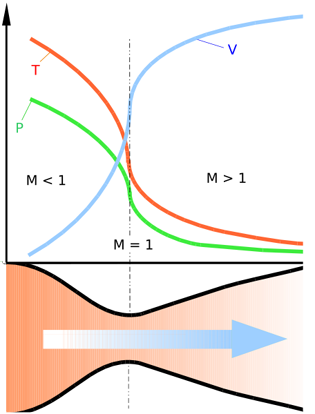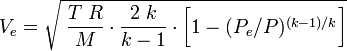De Laval nozzleBy Wikipedia,
the free encyclopedia,

http://en.wikipedia.org/wiki/De_Laval_nozzleDiagram of a de Laval nozzle, showing approximate flow velocity (v), together with the effect on temperature (t) and pressure (p)

A de Laval nozzle (or convergent-divergent nozzle, CD nozzle or con-di nozzle) is a tube that is pinched in the middle, making an hourglass-shape. It is used as a means of accelerating the flow of a gas passing through it to a supersonic speed. It is widely used in some types of steam turbine and is an essential part of the modern rocket engine and supersonic jet engines.

Similar flow properties have been applied to jet streams within astrophysics.

## History

The nozzle was developed by Swedish inventor Gustaf de Laval in 1897 for use on an impulse steam turbine.

This principle was used in a rocket engine by Robert Goddard, and very nearly all modern rocket engines that employ hot gas combustion use de Laval nozzles.

## Operation

Its operation relies on the different properties of gases flowing at subsonic and supersonic speeds. The speed of a subsonic flow of gas will increase if the pipe carrying it narrows because the mass flow rate is constant. The gas flow through a de Laval nozzle is isentropic (gas entropy is nearly constant). At subsonic flow the gas is compressible; sound, a small pressure wave, will propagate through it. At the "throat", where the cross sectional area is a minimum, the gas velocity locally becomes sonic (Mach number = 1.0), a condition called choked flow. As the nozzle cross sectional area increases the gas begins to expand and the gas flow increases to supersonic velocities where a sound wave will not propagate backwards through the gas as viewed in the frame of reference of the nozzle (Mach number > 1.0).

## Conditions for operation

A de Laval nozzle will only choke at the throat if the pressure and mass flow through the nozzle is sufficient to reach sonic speeds, otherwise no supersonic flow is achieved and it will act as a Venturi tube.

In addition, the pressure of the gas at the exit of the expansion portion of the exhaust of a nozzle must not be too low. Because pressure cannot travel upstream through the supersonic flow, the exit pressure can be significantly below ambient pressure it exhausts into, but if it is too far below ambient, then the flow will cease to be supersonic, or the flow will separate within the expansion portion of the nozzle, forming an unstable jet that may 'flop' around within the nozzle, possibly damaging it.

In practice ambient pressure must be no higher than roughly 2-3 times the pressure in the supersonic gas at the exit for supersonic flow to leave the nozzle.

## Analysis of gas flow in de Laval nozzles

The analysis of gas flow through de Laval nozzles involves a number of concepts and assumptions:

• For simplicity, the gas is assumed to be an ideal gas.
• The gas flow is isentropic (i.e., at constant entropy). As a result the flow is reversible (frictionless and no dissipative losses), and adiabatic (i.e., there is no heat gained or lost).
• The gas flow is constant (i.e., steady) during the period of the propellant burn.
• The gas flow is along a straight line from gas inlet to exhaust gas exit (i.e., along the nozzle's axis of symmetry)
• The gas flow behavior is compressible since the flow is at very high velocities.

## Exhaust gas velocity

As the gas enters a nozzle, it is traveling at subsonic velocities. As the throat contracts down the gas is forced to accelerate until at the nozzle throat, where the cross-sectional area is the smallest, the linear velocity becomes sonic. From the throat the cross-sectional area then increases, the gas expands and the linear velocity becomes progressively more supersonic.

The linear velocity of the exiting exhaust gases can be calculated using the following equation:$V_e = \sqrt{\;\frac{T\;R}{M}\cdot\frac{2\;k}{k-1}\cdot\bigg[ 1-(P_e/P)^{(k-1)/k}\bigg]}$

Some typical values of the exhaust gas velocity Ve for rocket engines burning various propellants are:

As a note of interest, Ve is sometimes referred to as the ideal exhaust gas velocity because it based on the assumption that the exhaust gas behaves as an ideal gas.

As an example calculation using the above equation, assume that the propellant combustion gases are: at an absolute pressure entering the nozzle of P = 7.0 MPa and exit the rocket exhaust at an absolute pressure of Pe = 0.1 MPa; at an absolute temperature of T = 3500 K; with an isentropic expansion factor of k = 1.22 and a molar mass of M = 22 kg/kmol. Using those values in the above equation yields an exhaust velocity Ve = 2802 m/s or 2.80 km/s which is consistent with above typical values.

The technical literature can be very confusing because many authors fail to explain whether they are using the universal gas law constant R which applies to any ideal gas or whether they are using the gas law constant Rs which only applies to a specific individual gas. The relationship between the two constants is Rs = R/M.

## Examples

For example a de Laval nozzle using hot air at a pressure of 1,000 psi (6.9 MPa or 68 atm), temperature of 1470 K, would have a pressure of 540 psi (3.7 MPa or 37 atm), temperature of 1269 K at the throat, and 15 psi (0.1 MPa or 1 atm), temperature of 502 K at the nozzle exit. The expansion ratio, nozzle cross sectional area at exit divided by area at throat, would be 6.8. The specific impulse would be 151 s (1480 N·s/kg).

### Application to celestial objects

Theoretical astrophysicists have found that pipes with the flow pattern of a De Laval nozzle have analogous phenomena in the interstellar medium. The interior of an accretion disk has a similar function as the pipe, save it is not a solid wall, but itself a fluid that can contain a relativistic jet by a pressure balanced boundary.

1. ^ Flack, Ronald D. (June 2005). Fundamentals of Jet Propulsion with Applications. Cambridge University Press. doi:10.2277/0521819830. ISBN 978-0521819831.
1.  Clarke, C. J. & Carswell B. (2007). Principles of Astrophysical Fluid Dynamics, chpt 9.2 (1st Edition ed.). Cambridge University Press. pp. 226. ISBN 978-0521853316.
2.  Richard Nakka's Equation 12
3.  Robert Braeuning's Equation 2.22
4.  Sutton, George P. (1992). Rocket Propulsion Elements: An Introduction to the Engineering of Rockets (6th Edition ed.). Wiley-Interscience. pp. 636. ISBN 0471529389.

Published in July 2009.